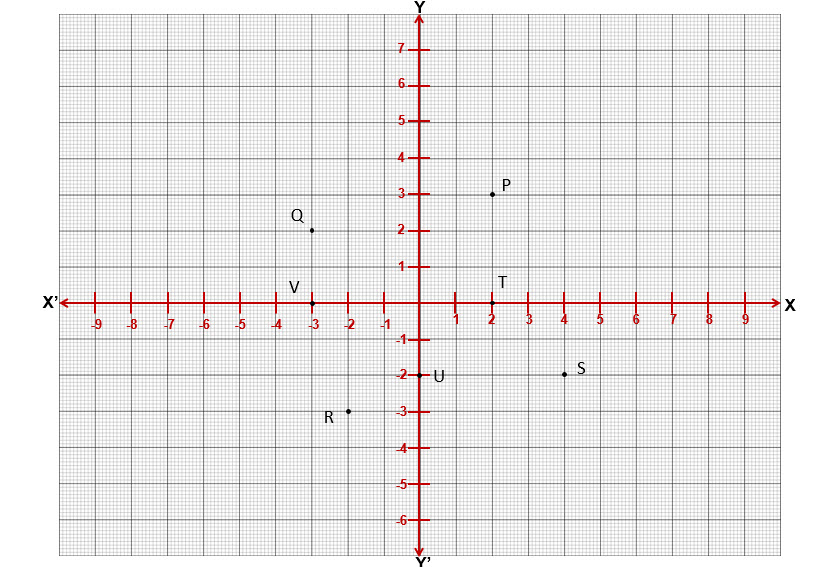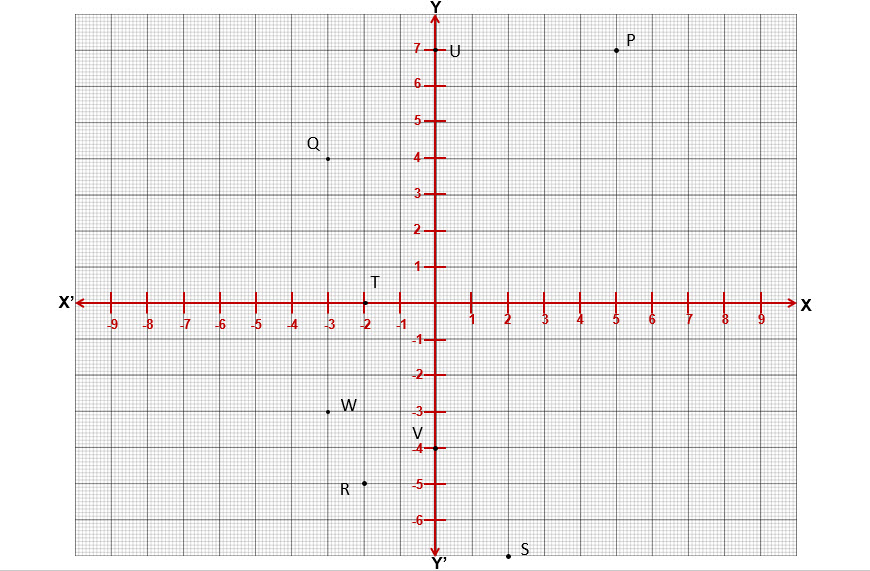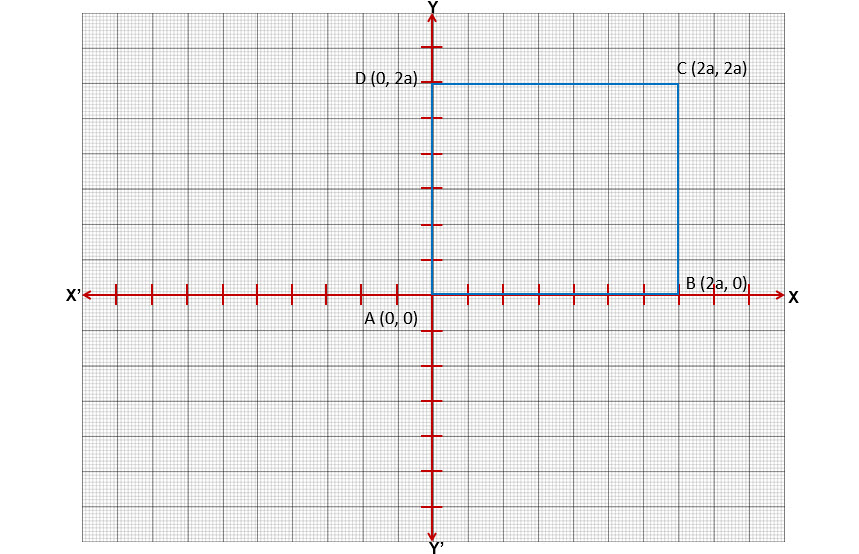Question 1: Write down the coordinates of each of the points$P, Q, R, S, T, U$ and$V$ shown in the adjoining graph.$P(2,3), Q(-3, 2), R(-2, -3), S(4, -2), Y(2, 0), V(-3, 0), U(0, -2)$$\\$

Question 2: Plot the following points in rectangular coordinate system. In which quadrant do they lie? i)$P (5, 7)$ (ii)$Q (-3, 4)$ (iii)$R (- 2, - 5)$ (iv)$S (2, - 7)$ (v)$T (- 2,0)$ (vi)$U(0,7)$ (vii)$V(0,-4)$ (vii)$W(-3,-3)$.$\\$

Question 3: On which axis do the following points lie? (i)$P (5,0)$ (ii)$Q (0, - 2)$ (iii)$R (- 4, 0)$ (iv)$S (0,5)$

(i)$P (5,0) - Lies \ on \ y-axis$           (ii)$Q (0, - 2) - Lies \ on \ x-axis$

(iii)$R (- 4, 0) - Lies \ on \ y-axis$      (iv)$S (0,5) - Lies \ on \ y-axis$$\\$

Question 4: Plot the points$(- 2, 0), (2, 0), (2, 2), (0, 4), (- 2, 2)$ and join them in order. What figure do you get?$\\$

Question 5: Let$ABCD$ be a square of side$2a$. Find the coordinates of the vertices of this Square when

(i)$A$ coincides with the origin and$AB$ and$AD$ are along$OX$ and$OY$ respectively.

(ii) The center of the square is at the origin and coordinate axes are parallel to the sides$AB$ and$AD$ respectively.

i)ii)$\\$

Question 6: The base$PQ$ of two equilateral triangles$PQR$ and$PQR'$ with side$2a$ lies along$Y$ axis such that the mid-point of$PQ$ is at the origin. Find the coordinates of the vertices$R$ and$R'$ of the triangles.$\\$

Question 7: Draw the quadrilateral whose vertices are:

(i)$P (1, 1), Q(2,4), R(8,4)$ and$S (10,1)$

(ii)$A (-2, 2), B (- 4, 2), C (- 6,- 2)$ and$D (- 4, - 2)$

(iii)$P (1, 4), Q(-5, 4), R(-5,- 3)$ and$S (1,-3)$

Name the type of quadrilateral so formed in each case.

i)

ii)

iii)$\\$
Question 8: Plot the points$O (0, 0), P (4,0)$ and$Q (2, 6)$ in a rectangular coordinate system. Mark midpoint of$OP$ as$L$. Write its coordinates. Draw the median of$\triangle OPQ$ passing through$Q$ and write the coordinates of its centroid.$\\$